Courses

# Electric Field due to a Dipole Notes | EduRev

## Class 12 : Electric Field due to a Dipole Notes | EduRev

The document Electric Field due to a Dipole Notes | EduRev is a part of the Class 12 Course Physics Class 12.
All you need of Class 12 at this link: Class 12

WHAT IS AN ELECTRIC DIPOLE
An electric dipole is defined as a pair of equal and opposite charges separated by a distance. However, a continuous charge distribution can also be approximated as an electric dipole from a large distance. These dipoles are characterized by their dipole moment, a vector quantity defined as the charge multiplied by their separation and the direction of this vector quantity is from the -Ve charge to the +ve charge. The total charge corresponding to a dipole is always zero. As the positive and negative charge centers are separated by a finite distance, the electric field at a test point does not cancel out completely leading to a finite electric field. Similarly, we also get finite electric potential due to a dipole.

ELECTRIC FIELD DUE TO A DIPOLE
The electric field due to a pair of equal and opposite charges at any test point can be calculated using the Coulomb’s law and the superposition principle. Let the test point P be at a distance r from the center of the dipole. The distance between +q and -q is d. We have shown the situation in the diagram below.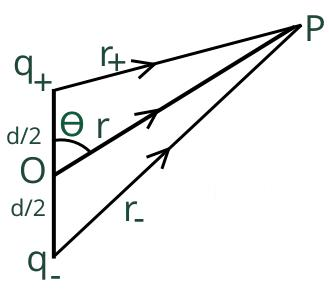I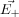an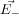be the electric field at point P due to the positive and the negative charges separately then the total electric fiel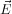at Point P can be calculated by using the superposition principle.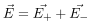Please note that the directions oandare alon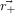and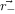respectively. This is the most general form of the electric field due to a dipole. However, we will express this vector in terms of radial and inclination vectors as shown in the diagram below.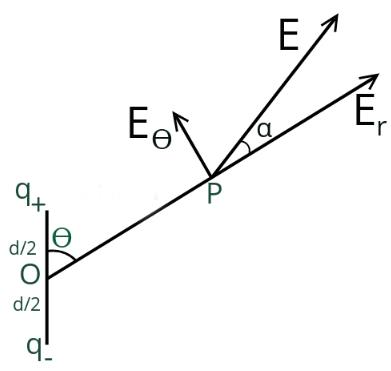In order to calculate the electric field in the polar coordinate, we will use the expression of the electric potential due to an electric dipole which we have calculated earlier.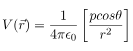Here p is the magnitude of the dipole moment and is given by qd

We can easily derive the electric field due to this dipole by calculating the negative gradient of this electric potential. In polar coordinate electric field will be independent of azimuthal (ϕ) coordinate.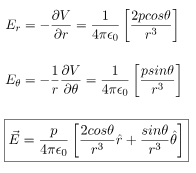The resultant electric field at point P is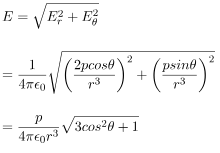As shown in the diagram, the resultant electric field makes an angle \alpha with the radial vector. Then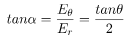ELECTRIC FIELD AT AN AXIAL POINT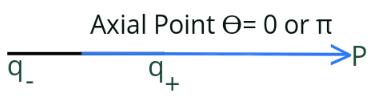In this case, the test point P is on the axis of the dipole. Consequently θ= 0 or π. The electric field at point P is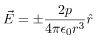SIMPLIFIED DERIVATION
The electric field at point P due to the positive charge is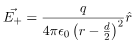Electric field at point P due to negative charge is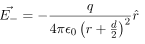Total electric field due to the dipole at axial point P is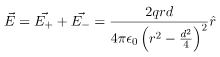At a relatively large distance r>>d/2 and we can approximate the electric field as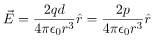ELECTRIC FIELD AT AN EQUATORIAL POINT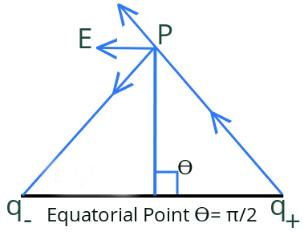In this case, the test point P is on the perpendicular bisector of the dipole. Consequently θ= π/2. The electric field at point P is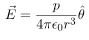SIMPLIFIED DERIVATION
The electric field at point P due to positive charge is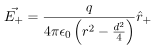Electric field at point P due to negative charge is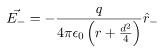Total electric field due to the dipole at equatorial point P is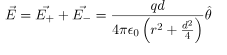At a relatively large distance r>>d/2 and we can approximate the electric field as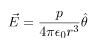Offer running on EduRev: Apply code STAYHOME200 to get INR 200 off on our premium plan EduRev Infinity!

,

,

,

,

,

,

,

,

,

,

,

,

,

,

,

,

,

,

,

,

,

;